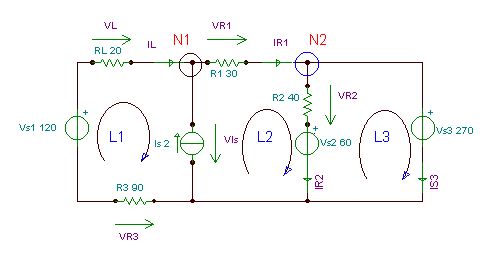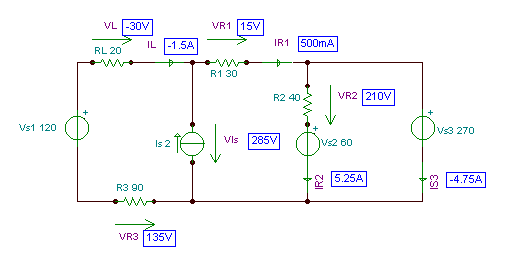# 基爾霍夫定律單擊或點擊下面的示例電路以調用TINACloud並選擇交互式DC模式以在線分析它們。I = VS /（R1+R2+R3）= 120 /（10 + 20 + 30）= 2 A.

V1= I * R1 = 2 * 10 = 20 V; V2 = I * R.2 = 2 * 20 = 40 V; V3 = I * R.3 = 2 * 30 = 60 V.- I1 - I2 - 一世3 = 0

I1 + I2 +我3 = 0

-V1+I1*R1-I3*R3 = 0

I3*R3 - 一世2*R2 +V2 = 0

I1+我2+我3 = 0 -8 + 40 * I1 - 40 *我3 = 0 40 * I3 -20 * I2 + 16 = 0

-V1 - （一世2 +我3）* R1 -I3*R3 = 0 or -8-（我2 +我3）* 40 - 我3* 40 = 0

I2 = –（V1 +我3*（R1+R3））/ R1 or I2 = - （8 + I3* 80）/ 40

I3*R3 + R2*（V1 +我3*（R1+R3））/ R1 +V2 = 0 or I3* 40 + 20 *（8 + I3* 80）/ 40 + 16 = 0

{TINA口譯員的解決方案}

I1 + I2 + I3 = 0
-V1+I1*R1-I3*R3=0
I3*R3-I2*R2+V2=0

I1 = [ - 50m]
I2 = [300m]
I3 = [ - 250m]
#Python解決方案

#我們有一個線性系統
#我們想要解決的方程：
#I1+I2+I3=0
#-V1+I1*R1-I3*R3=0
#I3*R3-I2*R2+V2=0

I1,I2,I3=s.symbols([‘I1′,’I2′,’I3’])
sol = s.solve([
I1+I2+I3,
-V1+I1*R1-I3*R3,
I3*R3-I2*R2+V2], [I1, I2, I3])

A= np.array([[1,1,1],[R1,0,-R3],[0,-R2,R3]])

b= np.array([0,V1,-V2])

x=np.linalg.solve(A,b)
#I1=x
#I2=x
#I3=x
#I1

#I2

#I3單擊/點擊上面的電路以在線分析，或單擊此鏈接以在Windows下保存

-IL +我R1 - 一世s = 0 （對於N1）

- 一世R1 +我R2 +我s3 = 0 （對於N2）

-Vs1 - V.R3 + V.Is + V.L = 0 （對於L1）

-VIs + V.s2 +VR2 +VR1 = 0 （對於L2）

-VR2 - V.s2 + V.s3 = 0 （對於L3）

VL = IL*RL

VR1 =IR1*R1

VR2 = IR2*R2

VR3 = –我L*R3

-IL +我R1 - 一世s = 0 （對於N1）

- 一世R1 +我R2 +我s3 = 0 （對於N2）

-Vs1 +我L*R3 + V.Is +我L*RL = 0 （對於L1）

-VIs + V.s2 +我R2*R2 +我R1*R1 = 0 （僅限 L2）

- 一世R2*R2 - V.s2 + V.s3 = 0 （對於L3）

-IL +我R1 - 一世s = 0 （對於N1）

- 一世R1 +我R2 +我s3 = 0 （對於N2）

-Vs1 +我L*R3 +我L*RL + V.s2 +我R2*R2 +我R1*R1 = 0 （L1）+（L2）

- 一世R2*R2 - V.s2 + V.s3 = 0 （對於L3）

-IL+IR1 - 2 = 0 （對於N1）

-IR1 +我R2 +我S3 = 0 （對於N2）

-120 – +我L* 90 +我L* 20 + 60 + I.R2* 40 +我R1* 30 = 0（L1）+（長2)

-IR2* 40 - 60 + 270 = 0 （對於L.3)

VR3 = –我L*R3= 135 V; VL = IL*RL = – 30 V； VIs = V.S1+VR3-VL = 285 V.

{TINA的口譯員對原始方程的求解}
Sys IL,IR1,IR2,Is3,VIs,VL,VR1,VR3,VR2
-IL-IS + IR1 = 0
-IR1 + IR2 + Is3 = 0
-Vs1 + VR3 +顯示-VL = 0
-Vis + VR1 + VR2 + Vs2 = 0
-Vs3 + VR2 + Vs2 = 0
VR1 = IR1 * R1
VR2 = IR2 * R2
VR3 = -IL * R3
VL = IL * RL

IL = [ - 1.5]
IR1 = [500m]
IR2 = [5.25]
Is3 = [ - 4.75]

VL = [ - 30]
VR1 = 
VR2 = 
VR3 = 
#Python解決方案
#Ax=b

#使用 numpy.solve 的符號解
#方程式：
#IL=-Is+IR1
#IR1=IR2+Is3
#Vs1+VR3-可見-VL=0
#Vis=VR1+VR2+Vs2
#Vs3=VR2+Vs2
#VR1=IR1*R1
#VR2=IR2*R2
#VR3=-IL*R3
#VL=IL*RL
#解決：
#IL,IR1,IR2,
#Is3，可見光，VL，
#VR1,VR3,VR2

IL,IR1,IR2,Is3,Vis,VL,VR1,VR3,VR2=s.symbols([‘IL’,’IR1′,’IR2′,’Is3′,’Vis’,’VL’,’VR1′,’VR3′,’VR2′])
sol = s.solve([
-Is+IR1-IL，
IR2+Is3-IR1,
Vs1+VR3-可見-VL，
VR1+VR2+Vs2-可見光，
VR2+Vs2-Vs3，
IR1*R1-VR1,IR2*R2-VR2,
-IL*R3-VR3,IL*RL-VL],[IL,IR1,IR2,Is3,Vis,VL,VR1,VR3,VR2])

#使用numpy.linalg解決的另一種方法
A=np.數組(
[[-1,1,0,0,0,0,0,0,0],
[0,-1,1,1,0,0,0,0,0],
[0,0,0,0,-1,-1,0,1,0],
[0,0,0,0,-1,0,1,0,1],
[0,0,0,0,0,0,0,0,1]
[0,R1,0,0,0,0,-1,0,0],
[0,0,R2,0,0,0,0,0,-1],
[-R3,0,0,0,0,0,0,-1,0],
[RL,0,0,0,0,-1,0,0,0]])

b=np.array([Is,0,-Vs1,-Vs2,Vs3-Vs2,0,0,0,0])

x=np.linalg.solve(A,b)

#IL=x IR1=x IR2=x
#Is3=x 可見=x VL=x
#VR1=x VR2=x VR3=x

print(“Is3= %.3f”%x)
print(“Vis=%.3f”%x)

 {TINA的口譯員對簡化的方程組的求解} 系統II，Ir1，Ir2，Is3 -il + Ir1-2 = 0 -Ir1 + Ir2 + Is3 = 0 -120+110*Il+60+40*Ir2+30*Ir1=0 -40 * Ir2 + 210 = 0 結束; IL = [ - 1.5] Ir1 = [500m] Ir2 = [5.25] Is3 = [ - 4.75]

 IL：= - 1.5; Ir1：= 0.5; Ir2：= 5.25; Is3：= - 4.75; VL：= 11 * RL; Vr1：= Ir1 * R1 Vr2：= Ir2 * R2; Vr3：= - 金正日* R3; 的VI：= Vs1-VL + Vr3; VL = [ - 30] Vr1 =  Vr2 =  Vr3 =  的VI =X很高興有你 DesignSoft
如果需要任何幫助找到合適的產品或需要支持，可以進行聊天。